# Robot Manipulation, Part 1: Kinematics

In this blog post, Sebastian Castro will talk about robot manipulation with MATLAB and Simulink. This part will discuss kinematics, and the next part will discuss dynamics.

– –

# Crash Course on Robot Manipulators

• Kinematics is the analysis of motion without considering forces. Here, we only need geometric properties such as lengths and degrees of freedom of the manipulator bodies.
• Dynamics is the analysis of motion caused by forces. In addition to geometry, we now require parameters like mass and inertia to calculate the acceleration of bodies.

Robot manipulators are often composed of several joints. Joints are composed of revolute (rotating) or prismatic (linear) degrees of freedom (DOF). Therefore, joint positions can be controlled to place the end effector of the robot in 3D space.

If you know the geometry of the robot and all its joint positions, you can do the math and figure out the position and orientation of any point on the robot. This is known as forward kinematics (FK).

The more frequent robot manipulation problem, however, is the opposite. We want to calculate the joint angles needed such that the end effector reaches a specific position and orientation. This is known as inverse kinematics (IK), and is more difficult to solve.

# Solving Inverse Kinematics

Depending on your robot geometry, IK can either be solved analytically or numerically.

• Analytic solutions mean that you can derive, in closed-form, an expression for the joint positions given the desired end effector position. This is beneficial because you do all the work offline and solving IK will be fast. As with everything in engineering: if you have an exact model of your system, you should take advantage of it!
• Numerical solutions are generally slower and less predictable than analytic solutions, but they can solve harder problems than analytic solutions (we expand on this below). However, these solutions introduce uncertainty in the form of initial conditions, optimization algorithm choice, or even random chance. So, you may not get the answer you want.

The 3D pose of your end effector can be specified by 6 parameters: 3 for position and 3 for orientation. Technically, you can derive an analytical solution if there are up to 6 nonredundant joints in your manipulator, assuming the desired position is reachable.

Robot designers have been clever about ensuring their manipulators have high degrees of freedom for controllability, while still ensuring analytical IK solutions are possible. For example, I have been taking the Udacity Robotics Software Engineer Nanodegree, where one of the projects involves analytic IK for a KUKA KR210 6-DOF manipulator. This manipulator has a spherical wrist that decouples the position and orientation analytical IK problems. You can find my writeup on GitHub.

So… why would you choose a numerical solution? Here are a few ideas.

• Your manipulator has redundant degrees of freedom (always the case with 7 or more)
• You don’t want to derive the math and have the computational resources for a numeric solution
• Your target position is not valid, but you still want to get as close to it as possible
• There are multiple, or even infinite, analytic solutions
• You want to introduce multiple, complex constraints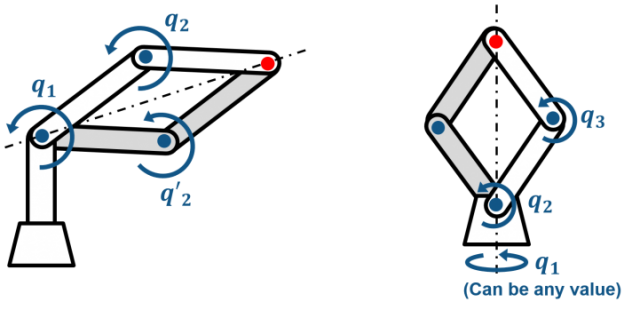Cases where there are multiple solutions, which are relatively easy to handle with analytical IK.
(Left) IK has exactly two solutions – “over” or “under”.
(Right) IK has infinite solutions since any rotation of the base is valid.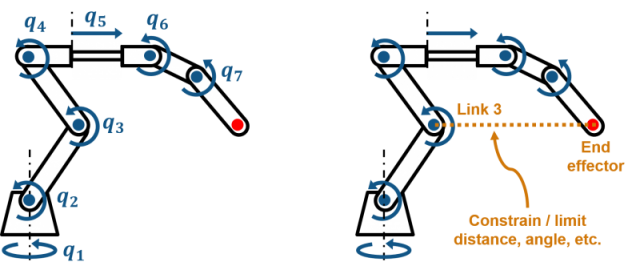Complex manipulation cases which are likely candidates for numerical solution.
(Left) 7-DOF manipulator can position the end effector with multiple valid solutions.
(Right) Example of position constraints between two coordinate frames on the manipulator.

To summarize, solving IK analytically is fast, accurate, and reliable. However, as you move towards more difficult problems, numerical solutions are often easier to implement, or even necessary.

# Representing Robot Manipulators in MATLAB and Simulink

Now, you hopefully have a basic idea of why manipulator kinematics are important, and what kind of real-world problems they can solve. There are two built-in ways you can work with robot manipulator models in MATLAB and Simulink.

## MATLAB

• How: Create a rigid body tree object
• When to use: Solving forward and inverse kinematics and dynamics, extracting mechanical properties (Jacobian, mass matrix, gravity torques, etc.)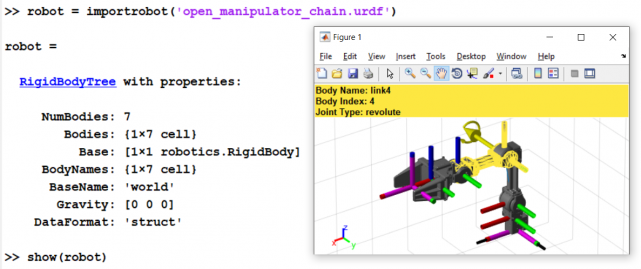• How: Create a Simscape Multibody model
• When to use: System-level dynamic simulation, integration with physical models of actuators, contact mechanics, etc.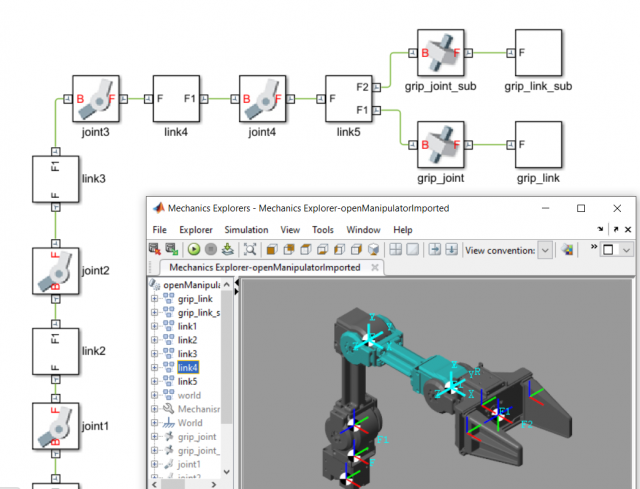Both rigid body tree objects and Simscape Multibody models can be created from scratch, or imported from Unified Robot Description Format (URDF) files. In addition, Simscape Multibody can also import 3D models from common CAD software. My colleague Christoph Hahn wrote a blog post on this.

Starting with release 2018a, Robotics System Toolbox includes a Manipulator Algorithms Simulink block library. These blocks allow you to perform kinematic and dynamic analysis on rigid body tree objects from Simulink, which makes the two representations above work together for system-level simulation and control design applications. You will learn more about this in Part 2.

… and yes, these blocks generate C/C++ code so you can deploy standalone algorithms outside of MATLAB and Simulink.

# Inverse Kinematics in MATLAB and Simulink

Robotics System Toolbox provides two numerical solvers for manipulator inverse kinematics:

• Inverse Kinematics: Enforces joint limits and lets you supply relative weights for each position and orientation target.
• Generalized Inverse Kinematics: Allows you to add multiple, and more complex, constraints such as relative position between coordinate frames, aiming at certain objects, or time-varying joint limits.

Below is some example MATLAB code and an animation of generalized IK on a model of a Rethink Sawyer, which has a 7-DOF arm. Here, we are setting a constraint on the end effector position, while simultaneously enforcing that the end effector points towards a separate target point near the ground.

sawyer = importrobot('sawyer.urdf', 'MeshPath', ...
fullfile(fileparts(which('sawyer.urdf')),'..','meshes','sawyer_pv'));
gik = robotics.GeneralizedInverseKinematics('RigidBodyTree',sawyer, ...
'ConstraintInputs',{'position','aiming'});

% Target Position constraint
targetPos = [0.5, 0.5, 0];
handPosTgt = robotics.PositionTarget('right_hand','TargetPosition',targetPos);

% Target Aiming constraint
targetPoint = [1, 0, -0.5];
handAimTgt = robotics.AimingConstraint('right_hand','TargetPoint',targetPoint);

% Solve Generalized IK
[gikSoln,solnInfo] = gik(sawyer.homeConfiguration,handPosTgt,handAimTgt)
show(sawyer,gikSoln);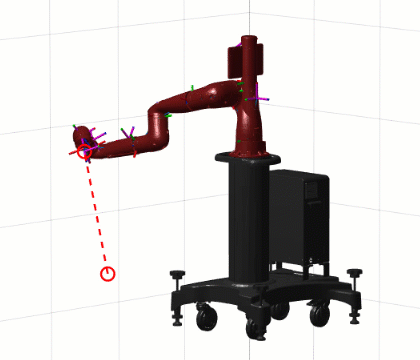What other constraints can you think of to make the motion smoother?

# Inverse Kinematics in the Bigger Picture

Once you’ve tested your IK solution, MATLAB and Simulink allow you to explore next steps towards building a complete robotic manipulation system, such as:

• Integrating IK with a simulation of the robot dynamics
• Adding other algorithms, such as supervisory logic, perception, and path planning
• Automatically generating standalone C/C++ code from your algorithms and deploying to hardware or middleware such as ROS

We discuss this in our video “Designing Robot Manipulator Algorithms “, which features the 4-DOF ROBOTIS OpenManipulator platform. You can download the example files from the MATLAB Central File Exchange.

# Conclusion

Many of you are likely developing algorithms for existing robots that already have built-in joint torque controllers. From this perspective, you can assume that the robot joints will adequately track any valid setpoint you provide.

Kinematics alone can be useful to design motion planning algorithms, as well as performing analysis based solely on robot geometry – for instance, workspace analysis or collision avoidance.

In the next part, we’ll talk more about manipulator dynamics and how this facilitates lower-level control design applications with MATLAB and Simulink.

Feel free to leave us a comment or email us at roboticsarena@mathworks.com. I hope you enjoyed reading!

– Sebastian

|